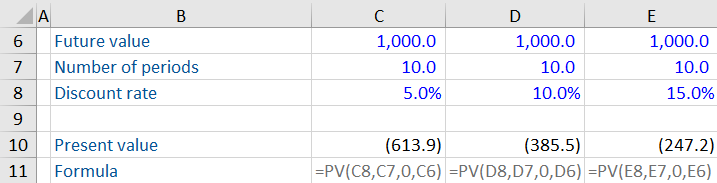## What is the Discount Rate?

The discount rate is the rate of return used to calculate the present value of a future cash flow. It is commonly used in a discounted cash flow analysis; a valuation technique that calculates the present value of a business’s forecasted free cash flows.

It is a key financial assumption which has many other uses in finance. These include the evaluation of various types of non-financial assets and liabilities:

• Employee benefits (defined pension benefit plans)
• Long-term provisions
• IFRS 16 Lease accounting
• Impairment of long-term cash-generating assets

All these examples require a discount rate to be determined. This can be difficult to determine, and differences in the rate can lead to variations in the present value amount calculated. In another context, the discount rate stands for the US Federal Reserve rate for loans given to banks. This blog focuses on the discount rate in the context of valuation and capital budgeting.

## Key Learning Points

• The discount rate is the rate of return used to calculate the present value (PV) of a future cash flow
• It is used to value various non-financial assets and liabilities including employee benefits, provisions, and lease accounting
• The WACC in a discounted cash flow analysis represents the discount rate which is used to discount the future cash flows to today’s value

## Discount rate applications

### Net present value

Net present value (NPV) is one of the most important concepts in valuation and capital budgeting. The NPV helps to evaluate investment opportunities by comparing the present value of cash outflows and inflows. A discount rate is used to calculate the NPV of a series of future cash payments.

### Discounted cash flow valuation

The discounted cash flow (DCF) valuation technique is used to calculate the present value of a business based on its forecasted free cash flows.  DCF involves several steps, including forecasting the future cash flows, calculating the WACC and the terminal value, and discounting the future cash flows to today. The WACC represents the discount rate. The sum of the discounted cash flows represents the enterprise value of the business.

### Perpetuity

A perpetuity is a repeated payment of an identical amount that is made in infinity. In valuation, the discount rate helps calculate the present value of an endless stream of cash flows.

### Discount rates in IFRS standards

standards use the discount rate to calculate the present value of future cash flows. These include:

• IAS 19 (Employee benefits)
• IAS 36 (Impairment of assets)
• IAS 37 (Provisions, contingent liabilities, and contingent assets)
• IFRS 13 Fair value measurement

An example is reporting of lease liabilities. Here, the amount reported in the balance sheet depends on the present value of future cash flows a company will pay over the life of the lease. Therefore, the discount rate is an important component to make sure this value is recorded accurately in a company’s balance sheet.

Another example is a defined benefit pension plan that guarantees compensation for employees in retirement. In such plans, the sponsoring employer must set aside funds today to meet the future final obligations. A discount rate helps in discounting the projected future cash outflows and estimating today’s value.

## Example: Calculate the present value based on the annual return (discount rate) to investors

An investment scheme will give a return of 1,000 after 10 years. We understand the role of the discount rate in calculating the present value of this investment.As the discount rate increases (from 5% to 15%), the present value of the future cash flow decreases. A higher rate of return results in investors getting the same return after 10 years by investing a lower sum today. Based on the discount rates, we can assume that investment 3 is the riskiest, and investment 1 is the safest among the three options.

## What is an Appropriate Discount Rate in the Context of DCF?

The discount rate used to determine the present value varies by situation. Here are a couple of factors that determine the appropriate discount rate in the context of DCF.

### Risk-free rate

This is the rate of return an investor would expect over time by investing in a risk-free investment. An example of a risk-free investment is US treasury bonds because the US government backs them. Theoretically, investors expect the risk-free rate as a minimum return on their investment.

### Weighted average cost of capital (WACC)

calculates a firm’s total cost of capital based on the weights and costs of all sources of capital. Many companies use WACC as the discount rate because it represents the minimum returns expected by all investors. If a business or project is riskier than the normal operations, investors may add a risk premium to WACC.